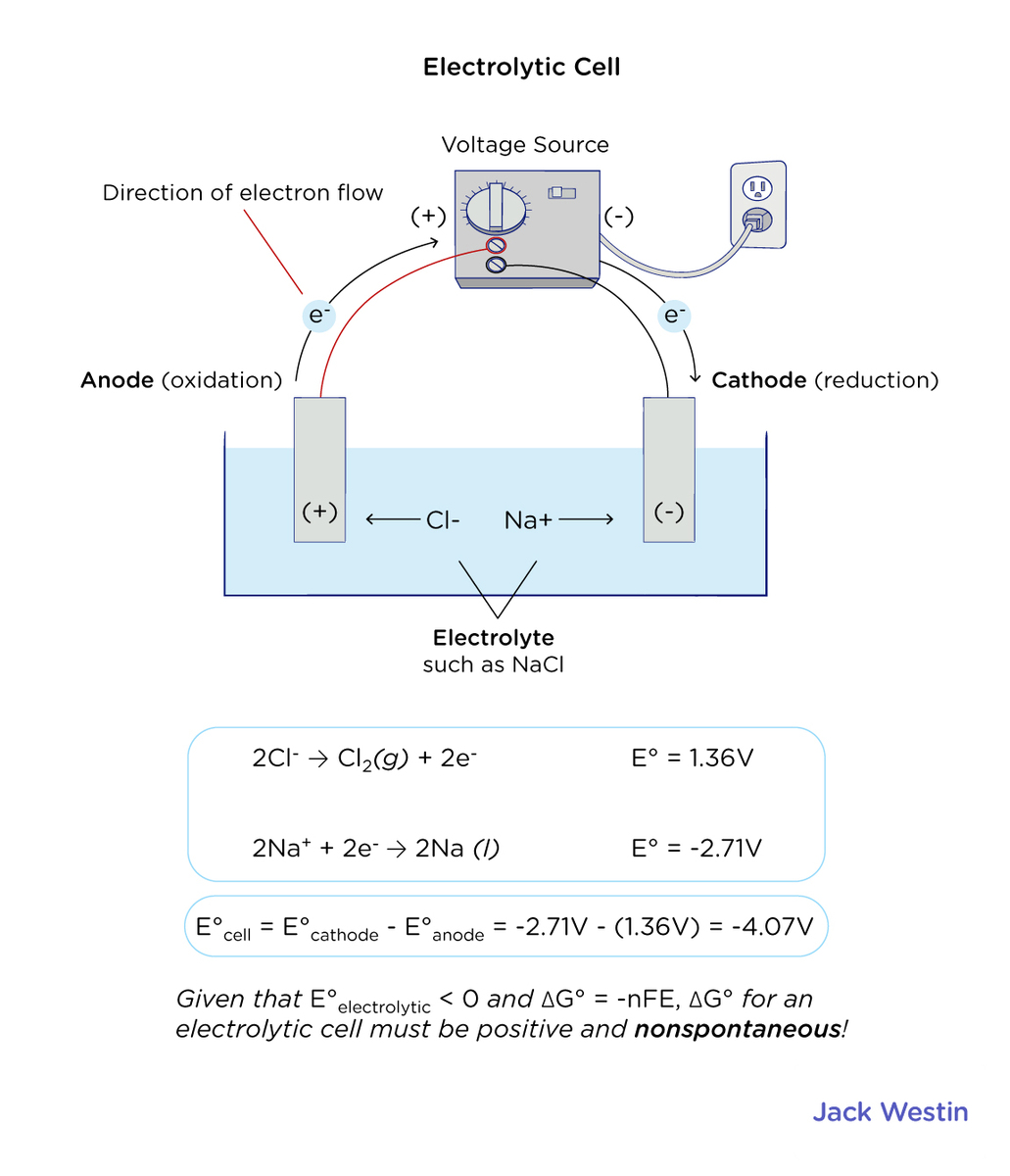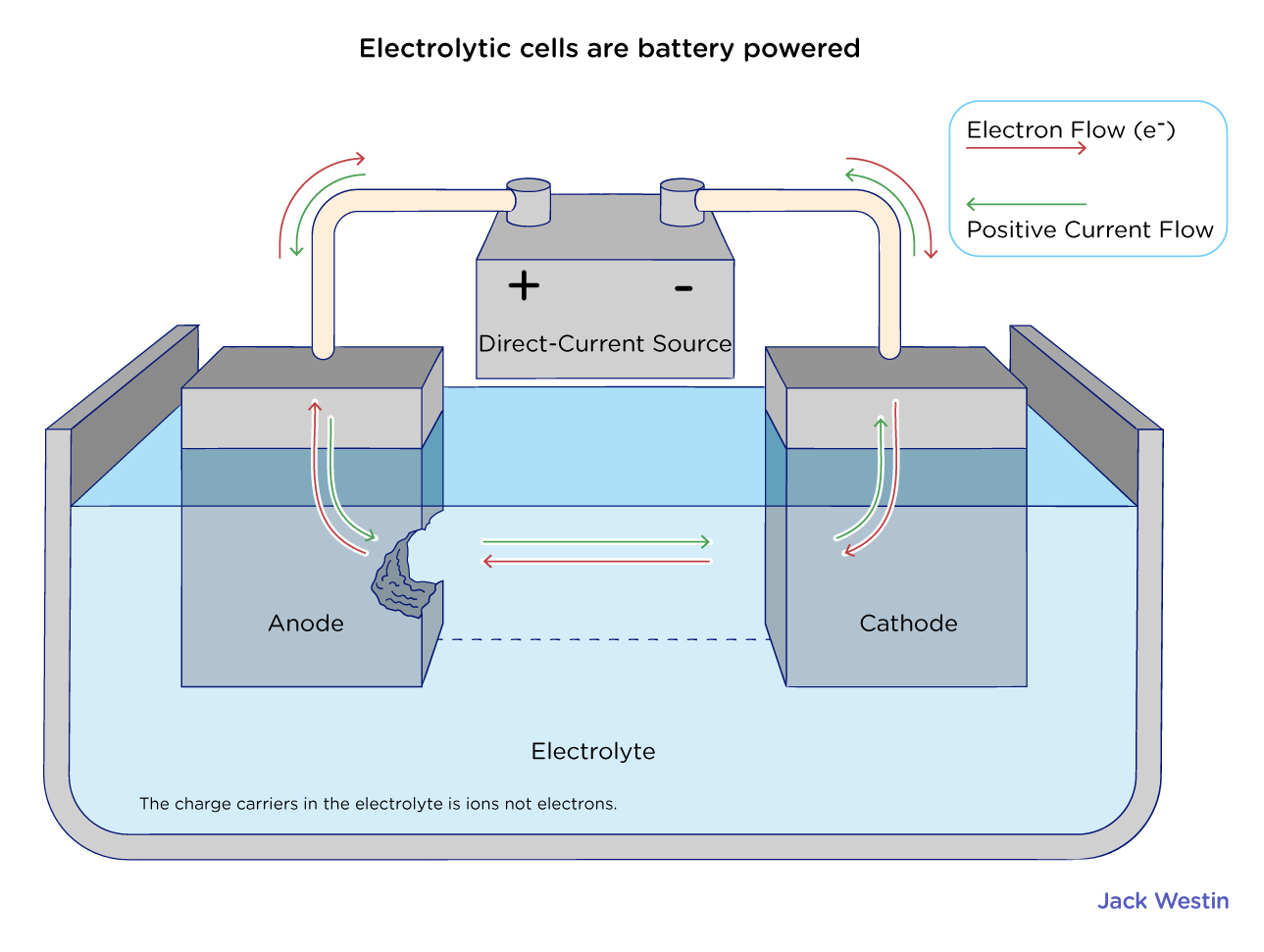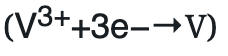Cyber Sale - Only 3 Days Remaining: ALL MCAT Products & Admissions Services

MCAT Content / Electrochemistry / Electrolytic Cell

### Electrolytic Cell

Topic: Electrochemistry

The main components of an electrolytic cell are an electrolyte, DC current, and two electrodes.Electrolysis

In order to predict the products of electrolysis, we first need to understand what electrolysis is and how it works. Electrolysis is a method of separating bonded elements and compounds by passing an electric current through them. It uses a direct electric current (DC) to drive an otherwise non-spontaneous chemical reaction. Electrolysis is very important commercially as a stage in the separation of elements from naturally occurring sources, such as ores, using an electrolytic cell.

The main components required to achieve electrolysis are:

– An electrolyte: a substance containing free ions, which are the carriers of electric current in the electrolyte. If the ions are not mobile, as in a solid salt, then electrolysis cannot occur.
– A direct current (DC) supply: provides the energy necessary to create or discharge the ions in the electrolyte. Electric current is carried by electrons in the external circuit.
– Two electrodes: an electrical conductor that provides the physical interface between the electrical circuit providing the energy and the electrolyte.

The key process of electrolysis is the interchange of atoms and ions by the removal or addition of electrons to the external circuit. The required products of electrolysis are in a different physical state from the electrolyte and can be removed by some physical processes.Anode and Cathode

Each electrode attracts ions that are of the opposite charge. Positively charged ions, or cations, move toward the electron-providing cathode, which is negative; negatively charged ions, or anions, move toward the positive anode. You may have noticed that this is the opposite of a galvanic cell, where the anode is negative and the cathode is positive.

Electrolyte

At the electrodes, electrons are absorbed or released by the atoms and ions. Those atoms that gain or lose electrons become charged ions that pass into the electrolyte. Those ions that gain or lose electrons to become uncharged atoms separate from the electrolyte. The formation of uncharged atoms from ions is called discharging. The energy required to cause the ions to migrate to the electrodes, and the energy to cause the change in ionic state, is provided by the external source.

Oxidation and Reduction

Oxidation of ions or neutral molecules occurs at the anode, and reduction of ions or neutral molecules occurs at the cathode. Neutral molecules can also react at either electrode. Electrolysis reactions involving H+ ions are fairly common in acidic solutions. In alkaline water solutions, reactions involving hydroxide ions (OH) are common. The substances oxidized or reduced can also be the solvent, which is usually water, or the electrodes. It is possible to have electrolysis involving gases.

The extent of chemical change that occurs in an electrolytic cell is stoichiometrically related to the number of moles of electrons that pass through the cell. From the perspective of the voltage source and circuit outside the electrodes, the flow of electrons is generally described in terms of electrical current using the SI units of coulombs and amperes. It takes 96,485 coulombs to constitute a mole of electrons, a unit known as the faraday (F).

This relation was first formulated by Michael Faraday in 1832, in the form of two laws of electrolysis:

1. The weights of substances formed at an electrode during electrolysis are directly proportional to the quantity of electricity that passes through the electrolyte.
2. The weights of different substances formed by the passage of the same quantity of electricity are proportional to the equivalent weight of each substance.

The equivalent weight of a substance is defined as the molar mass divided by the number of electrons required to oxidize or reduce each unit of the substance. Thus, one mole of V3+ corresponds to three equivalents of this species, and will require three faradays of charge to deposit it as metallic vanadium.Most stoichiometric problems involving electrolysis can be solved without explicit use of Faraday’s laws. The “chemistry” in these problems is usually very elementary; the major difficulties usually stem from unfamiliarity with the basic electrical units:

– current (in amperes) is the rate of charge transport: 1 amp = 1 C/s.
– power (in watts) is the rate of energy production or consumption: 1 w = 1 J/s.
– 1 kilowatt-hour = 3600 J.

Practice Questions

MCAT Official Prep (AAMC)

Chemistry Online Flashcards Question 5

Chemistry Online Flashcards Question 22

Chemistry Question Pack Passage 14 Question 78

Chemistry Question Pack Passage 19 Question 111

Chemistry Question Pack Passage 20 Question 113

Practice Exam 1 C/P Section Passage 10 Question 53

Practice Exam 1 C/P Section Passage 10 Question 55

Key Points

• The main components of an electrolytic cell are an electrolyte, DC current, and two electrodes.

• The key process of electrolysis is the interchange of atoms and ions by the removal or addition of electrons to the external circuit.

• Oxidation of ions or neutral molecules occurs at the anode, and reduction of ions or neutral molecules occurs at the cathode.

• From the perspective of the voltage source and circuit outside the electrodes, the flow of electrons is generally described in terms of electrical current using the SI units of coulombs and amperes.

• It takes 96,485 coulombs to constitute a mole of electrons, a unit known as the faraday (F).

• The equivalent weight of a substance is defined as the molar mass divided by the number of electrons required to oxidize or reduce each unit of the substance.

Key Terms

Electrolyte: A substance that, in solution or when molten, ionizes and conducts electricity.

Electrolysis: The chemical change produced by passing an electric current through a conducting solution or a molten salt.

Anode: The electrode of an electrochemical cell at which oxidation occurs.

Cathode: The electrode of an electrochemical cell at which reduction occurs.

Coulombs: In the International System of Units, the derived unit of electric charge; the amount of electric charge carried by a current of 1 ampere flowing for 1 second. Symbol: C.

Faraday: The quantity of electricity required to deposit or liberate 1 gram equivalent weight of a substance during electrolysis; approximately 96,487 coulombs.

Billing Information